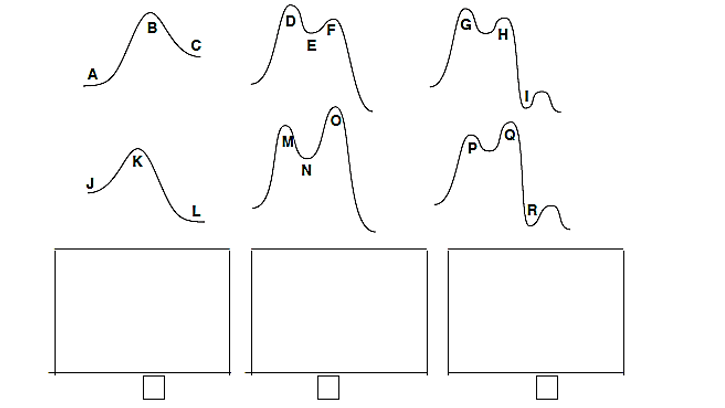# Problem: (1) CIRCLE the correct energy diagram below that represents the variation of energy vs. reaction coordinate for the SN1 reaction of 2-bromo-2-methylpropane with azide ion, N 3-,(2) DRAW the structure of the three species present at the points indicated on that diagram(3) INDICATE in the box below each structure which point (A-R) you are drawing. Use dotted lines and partial charges for transition states. (Notes: You need not draw out all the bonds within the N3-. Stereochemistry is not necessary.)

###### FREE Expert Solution
99% (304 ratings)
###### Problem Details

(1) CIRCLE the correct energy diagram below that represents the variation of energy vs. reaction coordinate for the SN1 reaction of 2-bromo-2-methylpropane with azide ion, N 3-,

(2) DRAW the structure of the three species present at the points indicated on that diagram

(3) INDICATE in the box below each structure which point (A-R) you are drawing. Use dotted lines and partial charges for transition states. (Notes: You need not draw out all the bonds within the N3-. Stereochemistry is not necessary.)Frequently Asked Questions

What scientific concept do you need to know in order to solve this problem?

Our tutors have indicated that to solve this problem you will need to apply the SN1 Reaction concept. You can view video lessons to learn SN1 Reaction. Or if you need more SN1 Reaction practice, you can also practice SN1 Reaction practice problems.

What is the difficulty of this problem?

Our tutors rated the difficulty of(1) CIRCLE the correct energy diagram below that represents ...as low difficulty.